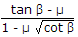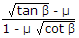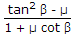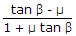# Civil Engineering - UPSC Civil Service Exam Questions

46.

If the flange angle is β, coefficient of friction between flange and rail is μ, the derailment coefficient according to Nadal's formula should not exceed

 A.B.C.D.Explanation:

No answer description available for this question. Let us discuss.

47.

Consider the following field tests :
4. Instrumented test pile
While estimating the load carrying capacity of a pile, the tests that can be used for separating the skin resistance from point resistance, would include

 A. 1 and 3 B. 1 and 4 C. 2 and 3 D. 2 and 4

Explanation:

No answer description available for this question. Let us discuss.

48.

Consider the following statements :
In water supply distribution network,
1. the grid-iron system requires more length of pipe lines and larger number of cut-off valves.
2. the design of the grid-iron system is difficult but economical.
3. employing a grid-iron system, the dead ends are completely eliminated.
4. employing a grid-iron system permits more water to be diverted towards the affected point from various directions.
Which of the statements given above are correct ?

 A. 1, 2 and 4 B. 1, 3 and 4 C. 2, 3 and 4 D. 1, 2 and 3

Explanation:

No answer description available for this question. Let us discuss.

49.

The entry of foul smelling gases into the house coming from the sewers can be prevented by

 A. providing water seals for all the fixtures B. providing water seals for all the fixtures and a vent pipe in the plumbing system C. providing sufficient vent pipes in the plumbing system D. exhaust fans

Explanation:

No answer description available for this question. Let us discuss.

50.

In the alignment of an irrigation channel wherefrom offtakes have to be provided at regular intervals, changes in the given channel parameters are made use of. The correct sequence of the decreasing order of preference of these parameters is

 A. Width, slope, depth B. Width, depth, slope C. Depth, slope, width D. Depth, width, slope